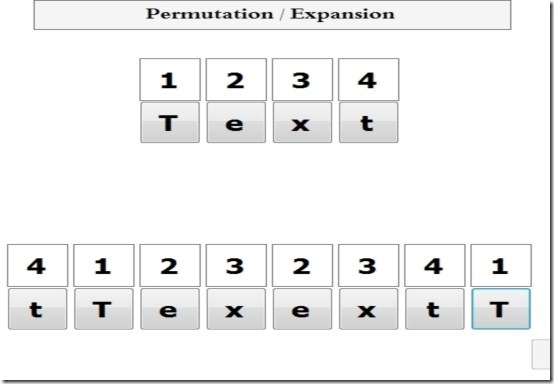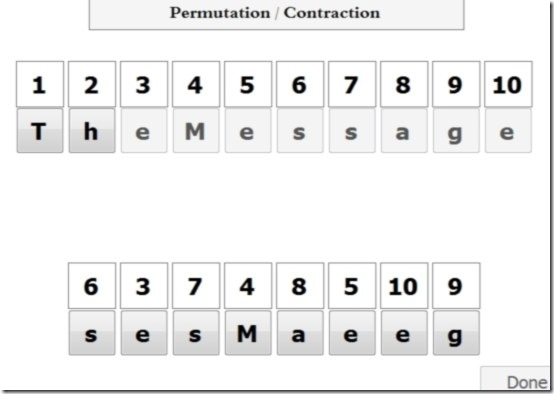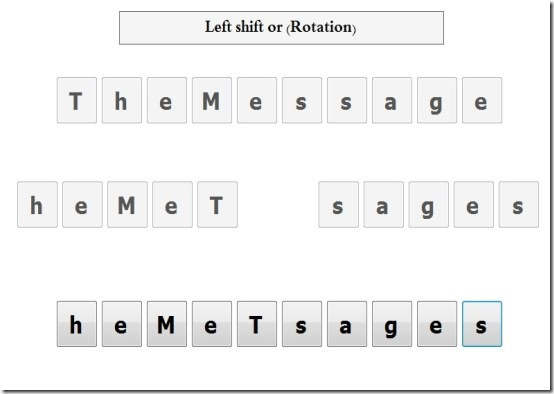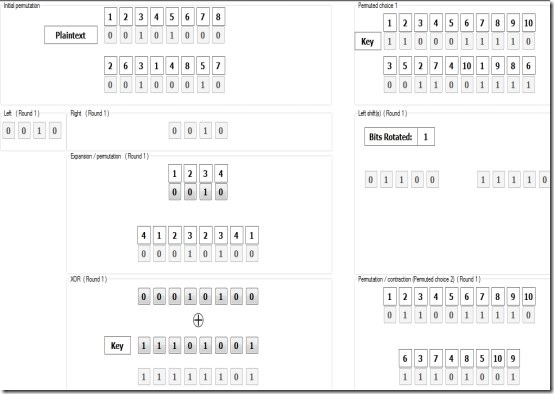Editor Ratings:
User Ratings:
[Total: 1 Average: 2]

DES Algorithm Teacher is a handy free software for learning about DES data encryption Algorithm. DES Algorithm Teacher teaches you about DES data encryption algorithm with the help of animations. It provides you a good overview of data encryption method. It explains you in detail about Simplified Data Encryption Standard (S-DES). DES Algorithm Teacher is easy to use freeware. It teaches you three different types of algorithms.

• General Algorithm
• Single Round Algorithm
• Full Algorithm

DES Algorithm Teacher includes a full example, which helps you gain a better understanding of algorithm. It basically explains in detail all the phases of encryption.

Here is a quick video of this software:

Let’s take a look at its main interface.## Using This Freeware to Learn and Understand Data Encryption Algorithm:

Download this freeware from the link given at the end of this review. It is downloaded in the form of a zip file. The size of zip file is only 84 KB. Extract this zip file into a folder on your PC. Installation is not required for this software. Just run its executable file and you are done.

DES Algorithm Teacher explains in detail all the encryption phases to you:

• Permutation
• Permutation/Expansion
• Permutation/Contraction
• Xor
• Substitute
• Left Shit

Permutation:  Permutation of a set of bits is an arrangement of those bits into a particular order. If the input is “TheMessage”, the output could be “eehsMeTgas”.Permutation/Expansion: In Permutation/Expansion, the input is expanded to 8 bits by using a permutation plus an expansion.  If the input is “Text”, the output could be “tTexextT”.Permutation/Contraction: In Permutation/Contraction, the input is compress 10 bits down to 8 bits using a permutation plus an contraction. If the input is “TheMessage”, the output could be “sesMaeeg”.Xor : In Xor,  the output will be o whenever both input are the same. The output will be 1 whenever both input differ.

Substitution : The substitution function consists of a set of two s-boxes, each of which accept 4 bits as input and produces two bits as output.

Left Shift :  In Left Shift, if the input is “TheMessage”, the output is “heMeTsages”.DES Algorithm Teacher explains all the above concepts using nice animation, so that you are able to easily understand how different phases of DES encryption work.

The best part is that DES Encryption also provides a full example that show input text and then all phases of encryption, so that you know how the complete algorithm works. So, now when you come across encryption software that show they use DES, you know exactly what it means.## Key Features of DES Algorithm Teacher:

• DES Algorithm Teacher explains you the process of data encryption using DES Algorithm with the help of animations.
• It is very small in size.
• Completely free.
• DES Algorithm Teacher is compatible with Windows XP/Vista/7/8/8.1.
• It is a portable software and does not need installation.
• DES Algorithm Teacher includes a full example for understanding the algorithm.
• It covers all the  phases of the encryption.

## My Opinion About DES Algorithm Teacher:

DES Algorithm Teacher is a cool and trendy freeware for learning and understanding data encryption algorithm. It is a portable tool and can be easily carried into a USB flash drive. DES Algorithm Teacher explains data encryption algorithm with the help of suitable animations. I like this software and recommend it all of my readers.